# Notebook 2.2- Weighted and directed graphs¶

In :
import networkx as nx
import matplotlib.pyplot as plt


In this notebook we will be showing how we can use NetworkX to study weighted and directed graphs. We will be building on the concepts that we followed in Notebook 2.1, and will therefore be reusing some of the code that we discussed there.

Given the amount of repetition on the sequence of commands that we will be using, we will take this opportunity to introduce the use of functions, optional function parameters, and conditional execution of function logic.

### Part 1 - Function Definitions¶

We will be jumping straight back into our usual graph - let us populate all its elements.

In :
G = nx.Graph()



In the previous notebook we arrived at a graph drawing style that seemed to be slightly better looking than the default one used by the nx.draw() command.

In :
nx.draw(G, with_labels = True, font_color = 'white', node_shape='s')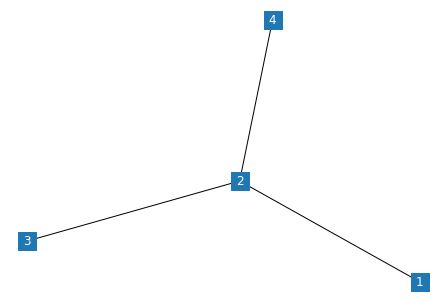As we are going to be using it quite often in this notebook, we can create our custom function, which we can use instead of the full command.

We shall name this show_graph().

In :
def show_graph():
nx.draw(G, with_labels = True, font_color = 'white', node_shape = 's')


Note that this function does not take any variables. When it comes to the graph that it is going to plot, it just uses whatever graph happens to be available in the variable G.

This is actually a very poor practice - if we happen to use another graph object, saved in a variable called G1, we wouldn't be able to draw it with the show_graph() command. We can overcome this limitation with the addition of a function parameter:

In :
def show_graph(graph):
nx.draw(graph, with_labels = True, font_color = 'white', node_shape = 's')

show_graph(G)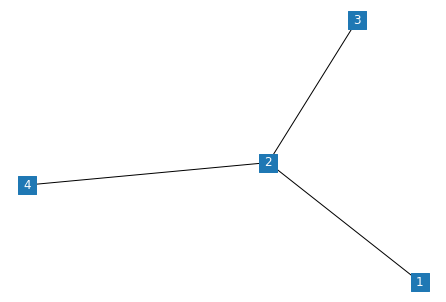Our parameter is called graph. If we now use the show_graph() function, we must also supply a graph object as a parameter. What would happen if we used it as before?

In :
show_graph()

---------------------------------------------------------------------------
TypeError                                 Traceback (most recent call last)
<ipython-input-12-cdd83e29cf16> in <module>
----> 1 show_graph()

TypeError: show_graph() missing 1 required positional argument: 'graph'

Python, very rightly, complaints! The error message very bluntly infroms us that an argument called 'graph' is missing.

We can have the best of the two worlds (convenience and customisation) using an optional parameter. In fact, this is what networkx does all of the time - you might have noticed that we can use nx.draw() with only one parameter or many more, and python never complaints!

In :
def show_graph(graph=G):
nx.draw(graph, with_labels = True, font_color = 'white', node_shape = 's')

show_graph()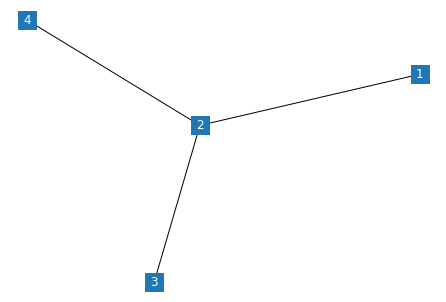In the revised function definition, we tell Python that this function can accept a parameter called graph, however if that is not supplied, it should just take whatever is stored in the variable called G. This will still fail, however, if there is no such variable, so make sure that the optional parameter assumptions you make are sensible!

Let's double check this with the Petersen graph generator, provided by networkx:

In :
show_graph(nx.petersen_graph())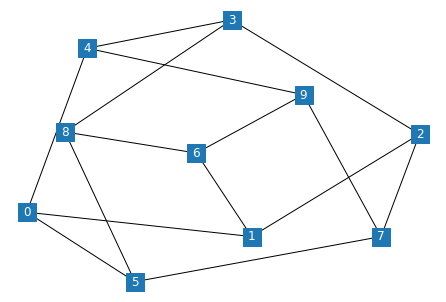Success! It all works as it should now. Let's continue by extending our original graph.

In :
G.add_edge(4,5)

show_graph()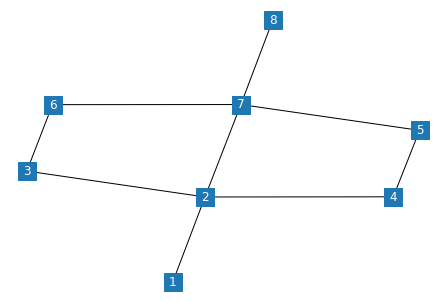Let us now see what a shortest path between nodes 1 and 8 would looks like. We will be reusing some of the code that we developed in the previous notebook.

In :
path = nx.shortest_path(G, source = 1, target =8)
edges_path = list(zip(path,path[1:]))

edges_path_reversed = [(y,x) for (x,y) in edges_path]
edges_path = edges_path + edges_path_reversed

edge_colors = ['black' if not edge in edges_path else 'red' for edge in G.edges()]

nx.draw(G, with_labels = True, font_color = 'white', edge_color= edge_colors, node_shape = 's')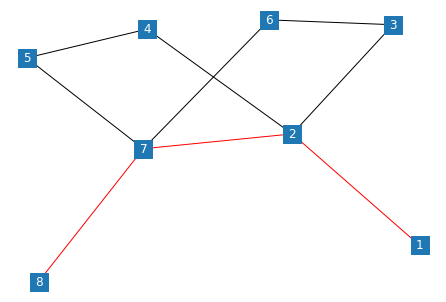Note that we have reused our edge reversion "hack" to overcome the issues that we encountered with bidirectionality, when it comes to highlighting the edges that are used by our path.

The highlighted edges definitely look useful, but wouldn't it be nice if we also highlighted the nodes? We can do this using the node_color parameter.

In :
path = nx.shortest_path(G, source = 1, target =8)
nodecol = ['steelblue' if not node in path else 'red' for node in G.nodes()]
nx.draw(G, with_labels = True, font_color = 'white', edge_color= edge_colors, node_shape = 's', node_color = nodecol)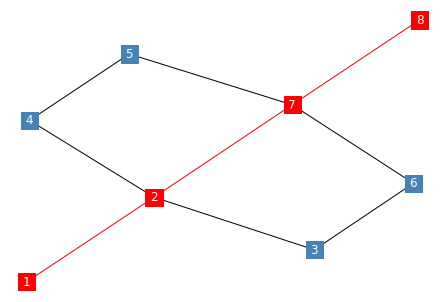Now, let's convert the above code into a function.

In :
def show_path(from_node, to_node):
path = nx.shortest_path(G, source = 1, target =8)

edges_path = list(zip(path,path[1:]))

edges_path_reversed = [(y,x) for (x,y) in edges_path]
edges_path = edges_path + edges_path_reversed
edge_colors = ['black' if not edge in edges_path else 'red' for edge in G.edges()]

nodecol = ['steelblue' if not node in path else 'red' for node in G.nodes()]
nx.draw(G, with_labels = True, font_color = 'white', edge_color= edge_colors, node_shape = 's', node_color = nodecol)

show_path(6, 4)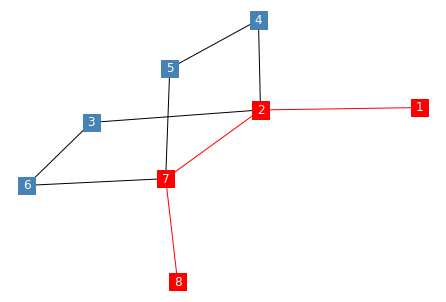### Part 2 - Weighted Graphs¶

We have so far assumed that our graphs are weightless - when it comes to calculating shortest paths, networkx uses a default weight for each edge.

We can inspect the parameter values of our edges using the following command:

In :
nx.get_edge_attributes(G,'weight')

Out:
{}

... nothing to see here!

It is to be expected, since we haven't actually assigned any weights so far. Let's initialise all weights to 1.

In :
for i,j in G.edges():
G[i][j]['weight'] = 1

nx.get_edge_attributes(G,'weight')

Out:
{(1, 2): 1,
(2, 3): 1,
(2, 4): 1,
(2, 7): 1,
(3, 6): 1,
(4, 5): 1,
(5, 7): 1,
(6, 7): 1,
(7, 8): 1}

Wonderful. We received a dictionary, with the edge values as a key and the weight as the value.

Now, how about plotting the weight of the edges alongside our graph?

In :
plt.figure()
pos = nx.spring_layout(G)
weight_labels = nx.get_edge_attributes(G,'weight')
nx.draw(G,pos,font_color = 'white', node_shape = 's', with_labels = True,)
nx.draw_networkx_edge_labels(G,pos,edge_labels=weight_labels)

Out:
{(1, 2): Text(-0.32453301987515465, -0.7018605194441813, '1'),
(2, 3): Text(0.010523033483430581, -0.1313706044061217, '1'),
(2, 4): Text(0.18018681496463956, -0.5195560128712517, '1'),
(2, 7): Text(-0.12646936954217883, -0.029980035230554647, '1'),
(3, 6): Text(0.18821408868471112, 0.43981402131137237, '1'),
(4, 5): Text(0.5195316737998721, -0.34915134332973086, '1'),
(5, 7): Text(0.2128754892930537, 0.1404246343109662, '1'),
(6, 7): Text(0.05122168565910171, 0.5412045904869394, '1'),
(7, 8): Text(-0.3832127426094284, 0.6111978414625396, '1')}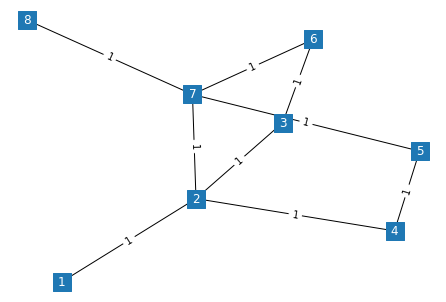Things begin to get a little bit messier. It turns out that our beloved nx.draw() command is not capable of plotting edge labels. To do this we need to use the nx.draw_networkx_edge_labels() function, which renders the edge values on top of a pre-existing drawing.

However, to make sure that the edge labels are rendered correctly, we need to fix position of the nodes. To do this, we use the nx.spring_layout() command, which automatically determines some positions for our graph - these are stored to the variable pos, and are then suplied to nx.draw() and nx.draw_networkx_edge_labels().

Let's now convert all of this into a new function, as we will be using it a lot more.

In :
def show_wgraph():
plt.figure()
pos = nx.spring_layout(G)
weight_labels = nx.get_edge_attributes(G,'weight')
nx.draw(G,pos,font_color = 'white', node_shape = 's', with_labels = True,)
output = nx.draw_networkx_edge_labels(G,pos,edge_labels=weight_labels)


We can now experiment with some weights.

In :
G['weight'] = 10

show_wgraph()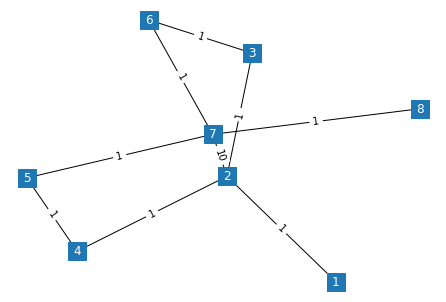And while we are at it, let's also implement our weighted shortest path function, incorporating our latest amendments to the rendering process.

In :
def show_wpath(from_node, to_node):
plt.figure()
pos = nx.spring_layout(G)

weight_labels = nx.get_edge_attributes(G,'weight')

path = nx.shortest_path(G, source = from_node, target = to_node)

edges_path = list(zip(path,path[1:]))
edges_path_reversed = [(y,x) for (x,y) in edges_path]
edges_path = edges_path + edges_path_reversed
edge_colors = ['black' if not edge in edges_path else 'red' for edge in G.edges()]

nodecol = ['steelblue' if not node in path else 'red' for node in G.nodes()]
nx.draw(G, pos, with_labels = True, font_color = 'white', edge_color= edge_colors, node_shape = 's', node_color = nodecol)
nx.draw_networkx_edge_labels(G,pos,edge_labels=weight_labels)

show_wpath(1,8)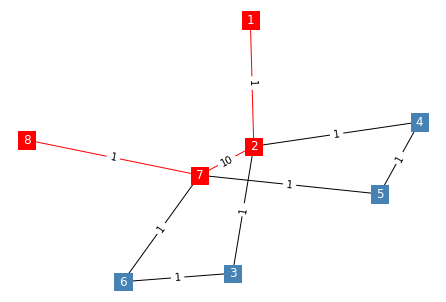There is something wrong here - the shortest path should definitely not pass from edge (2,7), given the weight of 10.

The issue actually lies with the nx.shortest_path() function - unless we explicitely tell it what weight values to consider, it assumes that no weights should be used.

To keep things simple, we will be using the nx.dijkstra_path() instead, which will use the weight parameters if they are present.

In :
def show_wpath_d(from_node, to_node):
plt.figure()
pos = nx.spring_layout(G)

weight_labels = nx.get_edge_attributes(G,'weight')

path = nx.dijkstra_path(G, source = from_node, target = to_node)

edges_path = list(zip(path,path[1:]))
edges_path_reversed = [(y,x) for (x,y) in edges_path]
edges_path = edges_path + edges_path_reversed
edge_colors = ['black' if not edge in edges_path else 'red' for edge in G.edges()]

nodecol = ['steelblue' if not node in path else 'red' for node in G.nodes()]
nx.draw(G, pos, with_labels = True, font_color = 'white', edge_color= edge_colors, node_shape = 's', node_color = nodecol)
nx.draw_networkx_edge_labels(G,pos,edge_labels=weight_labels)

show_wpath_d(1,8)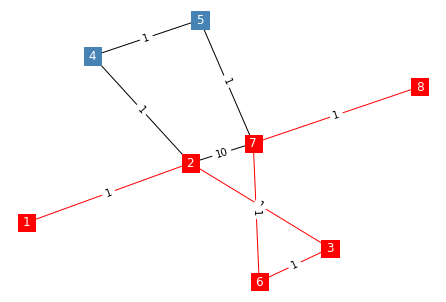### Part 3 - Weighted Graphs¶

In the final part of this notebook, we will focusing on the definition and manipulation of directed graphs.

We can initiate a directed graph object using the nx.DiGraph() class.

To get things started, we will seek to implement the directed graph that we used during when introducing Dijkstra's in our lecture .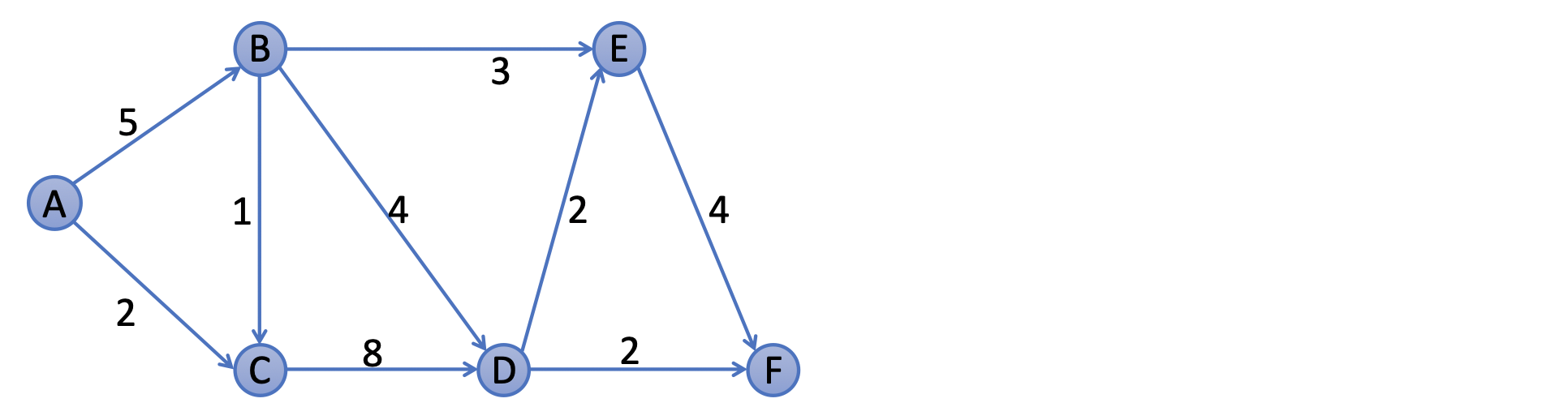As it happens, the G.add_edge() function contains a weight parameter which we can use to pass a value when we are declaring the edge. As you can see below the declaration process of the graph is exactly the same as before, with the exception of the use of DiGraph() class name when initialising the object.

In :
G = nx.DiGraph()

show_wgraph()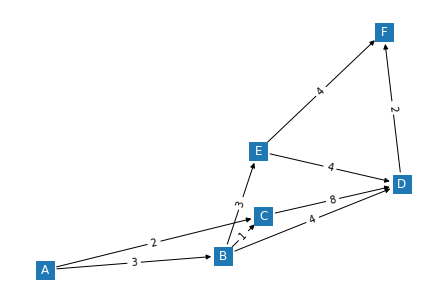As you can see above, our prevously defined show_wgraph() command is capable of visualising the direction of the edge without us having to add any new code - it appears that the nx.draw() function can tell the differnce between Graph and DiGraph.

Comment: I must admit that this is a neat little bonus, that I was not anticipating when I ran the command for the first time. In fact, I was bracing myself for having to add many more lines of code in our tired little function to make this happen.

The structure of the above graph looks OK, however, visually it does not look like the image that we used as a guide. This is becuase we have yet to define any locations for the nodes, and networkx uses its internal layout engine to come up with a random arrangement every time that we call the nx.draw() function.

To address this, we can define our own own position dictionary, and assign x,y coordinates on our own. We can assume that the nodes are positioned in a grid with 3 rows and 5 columns:

In :
node_pos = {'A':(1,2),'B':(2,1),'C':(2,3),'D':(3,3),'E':(4,1),'F':(5,3)}

nx.draw(G, node_pos)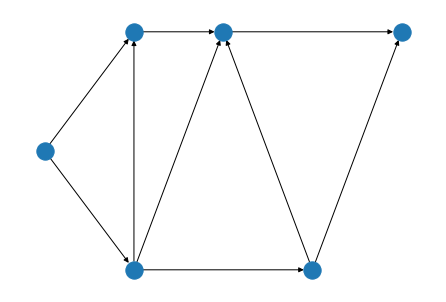Closer, but it is flipped vertically. It turns out that the 0 y coordinate lies at the top in the matplotlib world (remember, networkx uses matplotlib internally in order to draw the graphs).

In :
node_pos = {'A':(1,2),'B':(2,3),'C':(2,1),'D':(3,1),'E':(4,3),'F':(5,1)}

nx.draw(G, node_pos)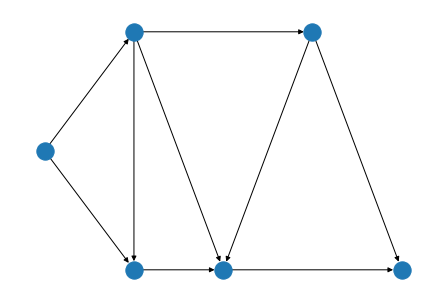We made it. Let's now update the show_wgraph() function to be able to accept a position parameter.

We will use an optional parameter value (as before) to capture the node positions, but this time we will assign it a default value of None (the value that Python uses for an empty value).

Our revised function will check whether the value is None - if it, it will invoke the nx.spring_layout() algorithm, alternatively it will use the supplied positions.

In :
def show_wgraph(custom_node_positions=None):
plt.figure()

if custom_node_positions==None:
pos = nx.spring_layout(G)
else:
pos=custom_node_positions

weight_labels = nx.get_edge_attributes(G,'weight')
nx.draw(G,pos,font_color = 'white', node_shape = 's', with_labels = True,)
output = nx.draw_networkx_edge_labels(G,pos,edge_labels=weight_labels)

show_wgraph(node_pos)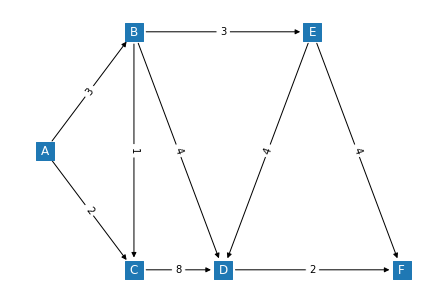Sucess! Let's double check that it still works without the parameter:

In :
show_wgraph()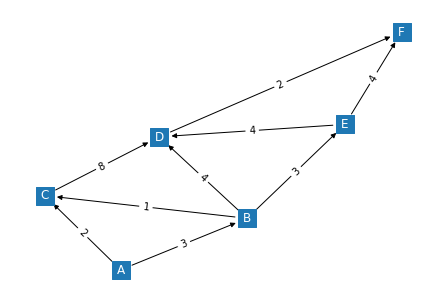Which is exactly as horrible as we anticipated.

One final bit for today - let's update the path visualisation function to check that networkx agrees with the "pen and paper" route that we obtained during the lecture:

In :
def show_wpath_d(from_node, to_node,custom_node_positions=None):
plt.figure()

if custom_node_positions==None:
pos = nx.spring_layout(G)
else:
pos=custom_node_positions

weight_labels = nx.get_edge_attributes(G,'weight')

path = nx.dijkstra_path(G, source = from_node, target = to_node)

edges_path = list(zip(path,path[1:]))
edges_path_reversed = [(y,x) for (x,y) in edges_path]
edges_path = edges_path + edges_path_reversed
edge_colors = ['black' if not edge in edges_path else 'red' for edge in G.edges()]

nodecol = ['steelblue' if not node in path else 'red' for node in G.nodes()]
nx.draw(G, pos, with_labels = True, font_color = 'white', edge_color= edge_colors, node_shape = 's', node_color = nodecol)
nx.draw_networkx_edge_labels(G,pos,edge_labels=weight_labels)

show_wpath_d('A','F',node_pos)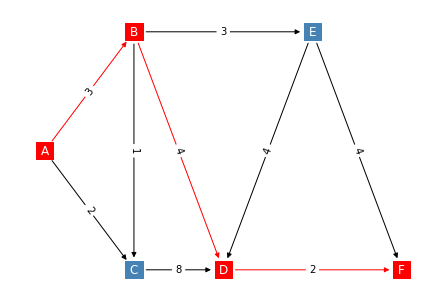Our job here is done.